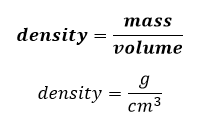# Problem: Iridium (Ir) has a face-centered cubic unit cell with an edge length of 383.3 pm. Calculate the density of solid iridium.

###### FREE Expert Solution

Recall that a Face-Centered Cubic (FCC) unit cell has 4 atoms per unit cell.

Formula for density:Given:

Edge length (a) = 383.3 pm

Molar mass of Iridium (Ir) = 192.2 g/mol (refer to the periodic table)

100% (302 ratings)###### Problem Details

Iridium (Ir) has a face-centered cubic unit cell with an edge length of 383.3 pm. Calculate the density of solid iridium.

Frequently Asked Questions

What scientific concept do you need to know in order to solve this problem?

Our tutors have indicated that to solve this problem you will need to apply the Unit Cell concept. You can view video lessons to learn Unit Cell. Or if you need more Unit Cell practice, you can also practice Unit Cell practice problems.

What professor is this problem relevant for?

Based on our data, we think this problem is relevant for Professor Harris' class at VCU.

What textbook is this problem found in?

Our data indicates that this problem or a close variation was asked in Chemistry: An Atoms First Approach - Zumdahl Atoms 1st 2nd Edition. You can also practice Chemistry: An Atoms First Approach - Zumdahl Atoms 1st 2nd Edition practice problems.